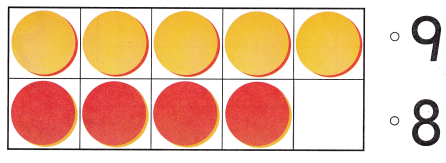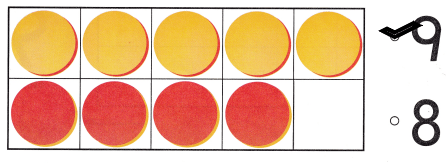# Texas Go Math Kindergarten Lesson 5.1 Answer Key Model and Count 9

Refer to our Texas Go Math Kindergarten Answer Key Pdf to score good marks in the exams. Test yourself by practicing the problems from Texas Go Math Kindergarten Lesson 5.1 Answer Key Model and Count 9.

## Texas Go Math Kindergarten Lesson 5.1 Answer Key Model and Count 9

Explore

DIRECTIONS: Model 8 objects. Show one more object. How many are there? Tell a friend how you know. Draw the objects.

Share and Show

DIRECTIONS: 1. Place counters as shown. Count and tell how many counters. 2. 5 are yellow. How many are red? Write the number. 3. Place counters in the ten frame to model nine. Trace the counters. Tell a friend what you know about the number 9.

Question 1.In the above ten-farme there are 5 yellow counters and 4 red counters.

Question 2.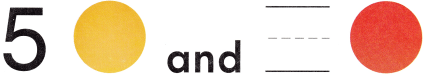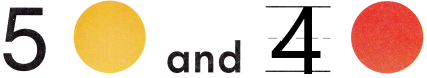Explanation:
There are 5 yellow counters and 4 red counters in the above ten-frame.So, i wrote the number 4 in the blank.

Question 3.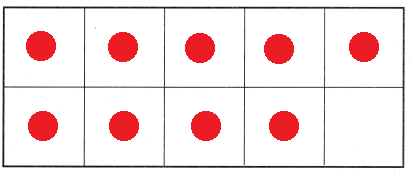Explanation:
I drew 9 counters to model the number 9.

DIRECTIONS: 4. Use two-color counters to model the different ways to make 9. Write to show some pairs of numbers that make 9.

Question 4.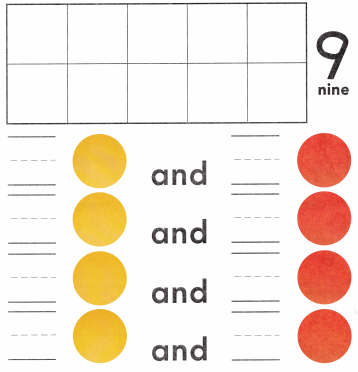Explanation:
I used two-color counters 7 yellow and 2 red counters to model the different ways to make 9 and i wrote to show some pairs of numbers that make 9, they are 7 and 2, 6 and 3, 5 and 4 and 4 and 5.

HOME ACTIVITY • Ask your child to show a set of eight objects. Have him or her show one more object and tell how many.

DIRECTIONS: 5. Count the flags in each set. Which sets show nine flags? Circle those sets. 6. Choose the correct answer. Which number does the model show?

Problem Solving

Question 5.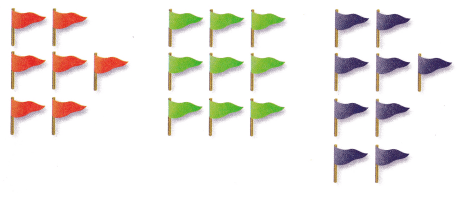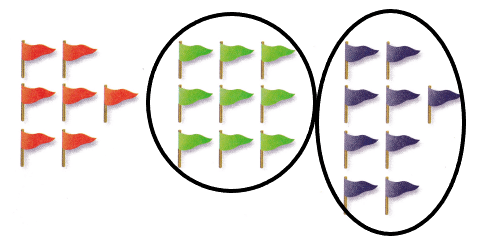Explanation:
I circled those groups of flags that are 9 in number.

Question 6.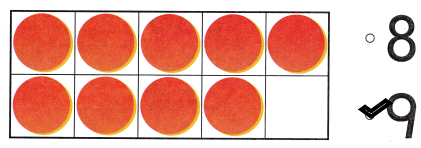Explanation:
I counted and marked the number 9 as there are 9 counters in the above ten-farme.

### Texas Go Math Kindergarten Lesson 5.1 Homework and Practice Answer Key

DIRECTIONS: 1. Draw some red and yellow counters to make 9 Write to show the numbers. 2. Draw some red and yellow counters to show another way to make 9. Write to show the numbers.

Question 1.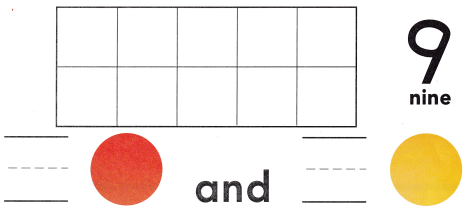Explanation:
I drew 3 red and 6 yellow counters to make 9 and wro the numbers 3 and 6.

Question 2.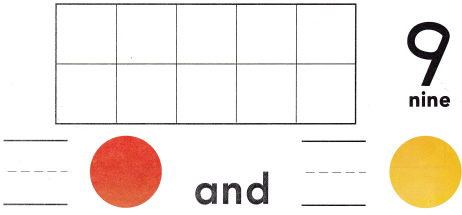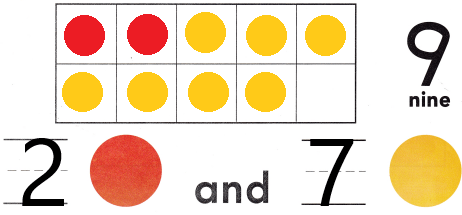Explanation:
I drew 2 red and 7 yellow counters to make 9 and wro the numbers 2 and 9.

DIRECTIONS: Choose the correct answer. 3-4. Which number does the model show?

Lesson Check

Question 3.Explanation:
I counted and marked the number 8 as there are 8 counters in the above ten-farme.

Question 4.